# mne.minimum_norm.make_inverse_operator#

mne.minimum_norm.make_inverse_operator(info, forward, noise_cov, loose='auto', depth=0.8, fixed='auto', rank=None, use_cps=True, verbose=None)[source]#

Assemble inverse operator.

Parameters:
info`mne.Info`

The `mne.Info` object with information about the sensors and methods of measurement. Specifies the channels to include. Bad channels (in `info['bads']`) are not used.

forward`dict`

Forward operator.

noise_covinstance of `Covariance`

The noise covariance matrix.

loose`float` | ‘auto’ | `dict`

Value that weights the source variances of the dipole components that are parallel (tangential) to the cortical surface. Can be:

• float between 0 and 1 (inclusive)

If 0, then the solution is computed with fixed orientation. If 1, it corresponds to free orientations.

• `'auto'` (default)

Uses 0.2 for surface source spaces (unless `fixed` is True) and 1.0 for other source spaces (volume or mixed).

• dict

Mapping from the key for a given source space type (surface, volume, discrete) to the loose value. Useful mostly for mixed source spaces.

depth

How to weight (or normalize) the forward using a depth prior. If float (default 0.8), it acts as the depth weighting exponent (`exp`) to use None is equivalent to 0, meaning no depth weighting is performed. It can also be a `dict` containing keyword arguments to pass to `mne.forward.compute_depth_prior()` (see docstring for details and defaults). This is effectively ignored when `method='eLORETA'`.

Changed in version 0.20: Depth bias ignored for `method='eLORETA'`.

fixed`bool` | ‘auto’

Use fixed source orientations normal to the cortical mantle. If True, the loose parameter must be “auto” or 0. If ‘auto’, the loose value is used.

rank`None` | ‘info’ | ‘full’ | `dict`

This controls the rank computation that can be read from the measurement info or estimated from the data. When a noise covariance is used for whitening, this should reflect the rank of that covariance, otherwise amplification of noise components can occur in whitening (e.g., often during source localization).

`None`

The rank will be estimated from the data after proper scaling of different channel types.

`'info'`

The rank is inferred from `info`. If data have been processed with Maxwell filtering, the Maxwell filtering header is used. Otherwise, the channel counts themselves are used. In both cases, the number of projectors is subtracted from the (effective) number of channels in the data. For example, if Maxwell filtering reduces the rank to 68, with two projectors the returned value will be 66.

`'full'`

The rank is assumed to be full, i.e. equal to the number of good channels. If a `Covariance` is passed, this can make sense if it has been (possibly improperly) regularized without taking into account the true data rank.

`dict`

Calculate the rank only for a subset of channel types, and explicitly specify the rank for the remaining channel types. This can be extremely useful if you already know the rank of (part of) your data, for instance in case you have calculated it earlier.

This parameter must be a dictionary whose keys correspond to channel types in the data (e.g. `'meg'`, `'mag'`, `'grad'`, `'eeg'`), and whose values are integers representing the respective ranks. For example, `{'mag': 90, 'eeg': 45}` will assume a rank of `90` and `45` for magnetometer data and EEG data, respectively.

The ranks for all channel types present in the data, but not specified in the dictionary will be estimated empirically. That is, if you passed a dataset containing magnetometer, gradiometer, and EEG data together with the dictionary from the previous example, only the gradiometer rank would be determined, while the specified magnetometer and EEG ranks would be taken for granted.

The default is `None`.

use_cps`bool`

Whether to use cortical patch statistics to define normal orientations for surfaces (default True).

verbose

Control verbosity of the logging output. If `None`, use the default verbosity level. See the logging documentation and `mne.verbose()` for details. Should only be passed as a keyword argument.

Returns:
invinstance of `InverseOperator`

Inverse operator.

Notes

For different sets of options (loose, depth, fixed) to work, the forward operator must have been loaded using a certain configuration (i.e., with force_fixed and surf_ori set appropriately). For example, given the desired inverse type (with representative choices of loose = 0.2 and depth = 0.8 shown in the table in various places, as these are the defaults for those parameters):

Inverse desired

Forward parameters allowed

loose

depth

fixed

force_fixed

surf_ori

Loose constraint,
Depth weighted

0.2

0.8

False

False

True

Loose constraint

0.2

None

False

False

True

Free orientation,
Depth weighted

1.0

0.8

False

False

True

Free orientation

1.0

None

False

False

True | False

Fixed constraint,
Depth weighted

0.0

0.8

True

False

True

Fixed constraint

0.0

None

True

True

True

Also note that, if the source space (as stored in the forward solution) has patch statistics computed, these are used to improve the depth weighting. Thus slightly different results are to be expected with and without this information.

For depth weighting, 0.8 is generally good for MEG, and between 2 and 5 is good for EEG, see .

References

## Examples using `mne.minimum_norm.make_inverse_operator`#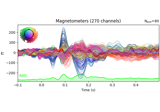Working with CTF data: the Brainstorm auditory dataset

Working with CTF data: the Brainstorm auditory dataset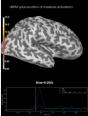Source localization with MNE, dSPM, sLORETA, and eLORETA

Source localization with MNE, dSPM, sLORETA, and eLORETA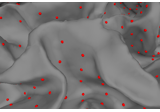The role of dipole orientations in distributed source localization

The role of dipole orientations in distributed source localization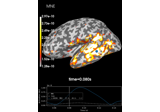Computing various MNE solutions

Computing various MNE solutions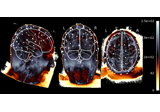EEG source localization given electrode locations on an MRI

EEG source localization given electrode locations on an MRI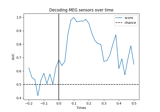Decoding (MVPA)

Decoding (MVPA)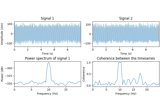DICS for power mapping

DICS for power mapping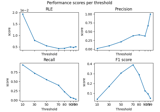Compare simulated and estimated source activity

Compare simulated and estimated source activity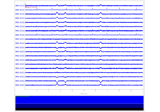Simulate raw data using subject anatomy

Simulate raw data using subject anatomy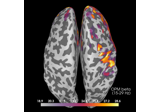Compute source power spectral density (PSD) of VectorView and OPM data

Compute source power spectral density (PSD) of VectorView and OPM data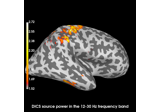Compute evoked ERS source power using DICS, LCMV beamformer, and dSPM

Compute evoked ERS source power using DICS, LCMV beamformer, and dSPM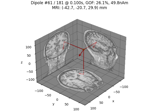Compute sparse inverse solution with mixed norm: MxNE and irMxNE

Compute sparse inverse solution with mixed norm: MxNE and irMxNE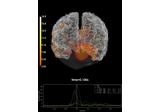Compute MNE inverse solution on evoked data with a mixed source space

Compute MNE inverse solution on evoked data with a mixed source space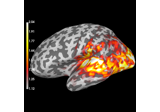Compute source power estimate by projecting the covariance with MNE

Compute source power estimate by projecting the covariance with MNE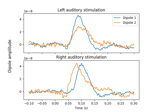Computing source timecourses with an XFit-like multi-dipole model

Computing source timecourses with an XFit-like multi-dipole model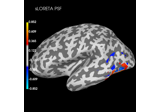Plot point-spread functions (PSFs) and cross-talk functions (CTFs)

Plot point-spread functions (PSFs) and cross-talk functions (CTFs)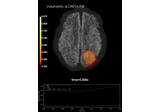Plot point-spread functions (PSFs) for a volume

Plot point-spread functions (PSFs) for a volume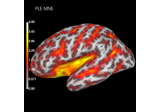Compute spatial resolution metrics in source space

Compute spatial resolution metrics in source space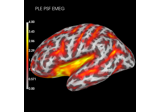Compute spatial resolution metrics to compare MEG with EEG+MEG

Compute spatial resolution metrics to compare MEG with EEG+MEG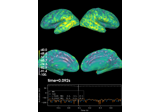Computing source space SNR

Computing source space SNR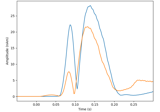Compute MxNE with time-frequency sparse prior

Compute MxNE with time-frequency sparse prior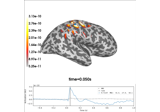Optically pumped magnetometer (OPM) data

Optically pumped magnetometer (OPM) dataFrom raw data to dSPM on SPM Faces dataset

From raw data to dSPM on SPM Faces dataset